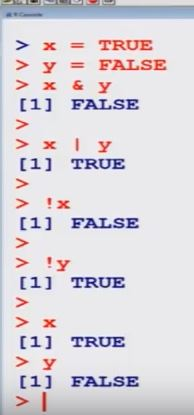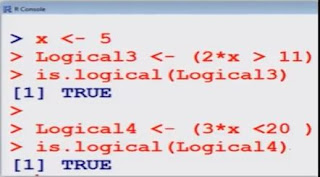# Basic calculations: Truth table and conditional executions

Example of standard logical operations

Truth table> x = TRUE
> y = FALSE

> x & y       # x AND y
   FALSE

> x | y        # x OR y
  TRUE

> !x          # negation of x
 FALSE

Example> x <- 5
> Logical1  <- (x > 2)
> is.logical (Logical1)
 TRUE

> Logical2 <- (x < 10)
> is.logical (Logical2)
 TRUE

Example> x <- 5
> Logical3 <-  (2*x > 11)
> is.logical (Logical3)
 TRUE

> Logical4  <-  (3*x <20)
> is.logical (Logical4)
 TRUE

Control structures in R :

control statements,
loops,
function
Conditional execution

1. Conditional execution

Syntax

if (condition) {executes commands if condition is TRUE}
if (condition) {executes commands if condition is TRUE}

else {executes commands if condition is FALSE}

• The condition in this control statement may not be vector valued and if so, only the first element of the vector is used.
• The condition may be complex expression where the logical operators "and" (&&) and "or" (| |) can be used.
Example

> x <- 5
> if ( x == 3)  { x <- x-1} else { x <- 2*x}
Interpretation:
• If x = 3, then execute  x = x - 1.
• If x ≠ 3, then execute x = 2*x.
In this case, x = 5 so x ≠ 3. Thus x = 2*5.> x
  10

Now choose x = 3 and repeat this example